Wave Optics

# The Wave Aspect of Light: Interference

OpenStaxCollege

### Learning Objectives

• Discuss the wave character of light.
• Identify the changes when light enters a medium.

We know that visible light is the type of electromagnetic wave to which our eyes respond. Like all other electromagnetic waves, it obeys the equation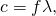where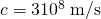is the speed of light in vacuum,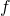is the frequency of the electromagnetic waves, and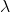is its wavelength. The range of visible wavelengths is approximately 380 to 760 nm. As is true for all waves, light travels in straight lines and acts like a ray when it interacts with objects several times as large as its wavelength. However, when it interacts with smaller objects, it displays its wave characteristics prominently. Interference is the hallmark of a wave, and in [link] both the ray and wave characteristics of light can be seen. The laser beam emitted by the observatory epitomizes a ray, traveling in a straight line. However, passing a pure-wavelength beam through vertical slits with a size close to the wavelength of the beam reveals the wave character of light, as the beam spreads out horizontally into a pattern of bright and dark regions caused by systematic constructive and destructive interference. Rather than spreading out, a ray would continue traveling straight ahead after passing through slits.

Making Connections: Waves

The most certain indication of a wave is interference. This wave characteristic is most prominent when the wave interacts with an object that is not large compared with the wavelength. Interference is observed for water waves, sound waves, light waves, and (as we will see in Special Relativity) for matter waves, such as electrons scattered from a crystal.

(a) The laser beam emitted by an observatory acts like a ray, traveling in a straight line. This laser beam is from the Paranal Observatory of the European Southern Observatory. (credit: Yuri Beletsky, European Southern Observatory) (b) A laser beam passing through a grid of vertical slits produces an interference pattern—characteristic of a wave. (credit: Shim’on and Slava Rybka, Wikimedia Commons)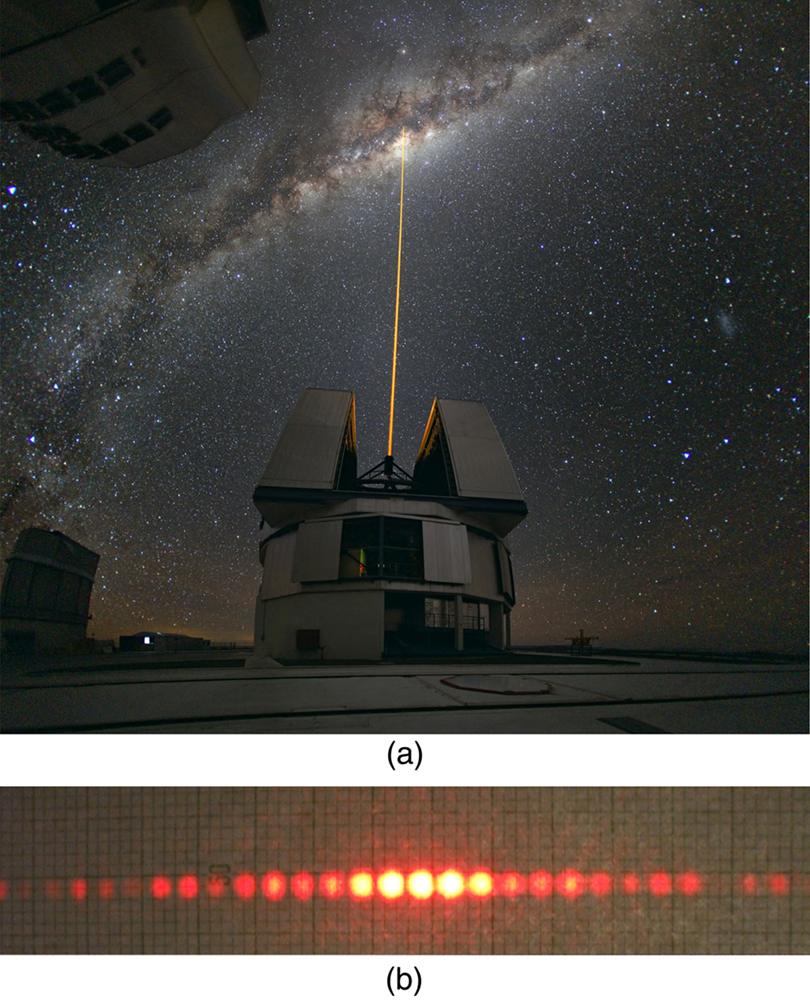Light has wave characteristics in various media as well as in a vacuum. When light goes from a vacuum to some medium, like water, its speed and wavelength change, but its frequencyremains the same. (We can think of light as a forced oscillation that must have the frequency of the original source.) The speed of light in a medium is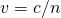, where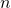is its index of refraction. If we divide both sides of equation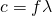by, we get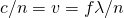. This implies that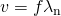, where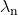is the wavelength in a medium and that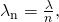whereis the wavelength in vacuum andis the medium’s index of refraction. Therefore, the wavelength of light is smaller in any medium than it is in vacuum. In water, for example, which has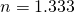, the range of visible wavelengths is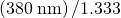to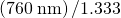, or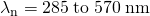. Although wavelengths change while traveling from one medium to another, colors do not, since colors are associated with frequency.

# Section Summary

• Wave optics is the branch of optics that must be used when light interacts with small objects or whenever the wave characteristics of light are considered.
• Wave characteristics are those associated with interference and diffraction.
• Visible light is the type of electromagnetic wave to which our eyes respond and has a wavelength in the range of 380 to 760 nm.
• Like all EM waves, the following relationship is valid in vacuum:, whereis the speed of light,is the frequency of the electromagnetic wave, andis its wavelength in vacuum.
• The wavelengthof light in a medium with index of refractionis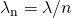. Its frequency is the same as in vacuum.

# Conceptual Questions

What type of experimental evidence indicates that light is a wave?

Give an example of a wave characteristic of light that is easily observed outside the laboratory.

# Problems & Exercises

Show that when light passes from air to water, its wavelength decreases to 0.750 times its original value.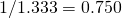Find the range of visible wavelengths of light in crown glass.

What is the index of refraction of a material for which the wavelength of light is 0.671 times its value in a vacuum? Identify the likely substance.

1.49, Polystyrene

Analysis of an interference effect in a clear solid shows that the wavelength of light in the solid is 329 nm. Knowing this light comes from a He-Ne laser and has a wavelength of 633 nm in air, is the substance zircon or diamond?

What is the ratio of thicknesses of crown glass and water that would contain the same number of wavelengths of light?

0.877 glass to water

## Glossary

wavelength in a medium, whereis the wavelength in vacuum, andis the index of refraction of the medium